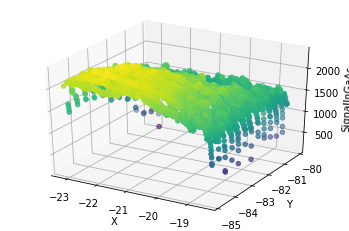# Data Illustration for the 2021 QSR Data Challenge Competition¶

We provide illustrative code that demonstrate how the data for the 2021 QSR Data Challenge Competition can be accessed in Python.

The data for each layer are provided in the HDF5 format, and can be accessed at the following link:

To access the variables in the dataset in Python, we utilize the h5py module. Details for installing this module are provided at the following link:

Quick Start Guide - h5py 3.2.1 documentation

Once the module has been installed and loaded, we read the data into Python by means of the h5py function. The File object that results is the starting point. There is only one data set that is stored in this File object, and its name is "OpenData".

In :
import h5py

sample_dataset_object = h5py.File('DATA_PORTION_layer352.hdf5', 'r')
list(sample_dataset_object.keys())

Out:
['OpenData']

We proceed to examine the data set as a Dataset object.

In :
sample_dataset = sample_dataset_object['OpenData'][:]

sample_dataset = sample_dataset.transpose()

sample_dataset[0:6][:]

Out:
array([[-2.2719162e+01, -8.0474930e+01,  4.8000000e+03,  1.5000000e+03,
1.0000000e+02,  0.0000000e+00,  1.6600000e+02,  1.0000000e+00,
9.0000000e+00],
[-2.2728518e+01, -8.0473801e+01,  4.8000000e+03,  1.5000000e+03,
1.0000000e+02,  1.4550000e+03,  1.6600000e+02,  1.0000000e+00,
9.0000000e+00],
[-2.2738997e+01, -8.0473427e+01,  4.8000000e+03,  1.5000000e+03,
1.0000000e+02,  3.1140000e+03,  4.7900000e+02,  1.0000000e+00,
9.0000000e+00],
[-2.2751347e+01, -8.0473053e+01,  4.8000000e+03,  1.5000000e+03,
1.0000000e+02,  4.0240000e+03,  7.8000000e+02,  1.0000000e+00,
9.0000000e+00],
[-2.2751347e+01, -8.0473053e+01,  4.8000000e+03,  1.5000000e+03,
1.0000000e+02,  4.2990000e+03,  9.4200000e+02,  1.0000000e+00,
9.0000000e+00],
[-2.2779041e+01, -8.0471931e+01,  4.8000000e+03,  1.5000000e+03,
1.0000000e+02,  4.4290000e+03,  9.8900000e+02,  1.0000000e+00,
9.0000000e+00]], dtype=float32)

In the data matrix, the columns correspond to (from left to right)

• X,
• Y,
• NominalPower,
• NominalSpeed,
• NominalSpotDiameter,
• LaserPowerCurrent,
• SignalInGaAs,
• IDbulkLayer, and
• IDoocLayer.

We provide a scatterplot visualization of the X, Y, and SignalInGaAs variables.

In :
from mpl_toolkits import mplot3d
import matplotlib.pyplot as plt
import numpy as np

X_variable = sample_dataset[:,0]
Y_variable = sample_dataset[:,1]
SignalInGaAs = sample_dataset[:,6]

fig = plt.figure()
ax = plt.axes(projection='3d')
ax.scatter3D(X_variable, Y_variable, SignalInGaAs, c=SignalInGaAs)
ax.set_xlabel('X')
ax.set_ylabel('Y')
ax.set_zlabel('SignalInGaAs')

Out:
Text(0.5, 0, 'SignalInGaAs')